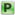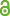Hauptmenü
• Autor
• Phan, Thanh Xuan
• Steinbach, Olaf
• TitelBoundary integral equations for optimal control problems with partial Dirichlet control
• Datei
• LicenceCC-BY
• Zugriffsrechte• Download Statistik295
• Peer ReviewJa
• AbstractIn this paper we study an optimal control problem where the Dirichlet control is considered on a part Γ D of the boundary Γ, while on the remaining part Γ\Γ D Neumann boundary conditions are given. Boundary integral operators are used to de- scribe the Steklov–Poincaré operator to realize the Dirichlet to Neumann map which is involved in both the primal and adjoint boundary value problem. In the case of box constraints on the control we have to solve a variational inequality in the Sobolev trace space H 1/2 (Γ D ). For the related Galerkin boundary element discretisation we present stability and error estimates, and we give some numerical examples.